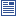Accessibility options:

# Matrices resources

Show me all resources applicable to

### Community Project (1)Differentiation for Economics and Business Studies Functions of Multi-Variable Functions (SOURCE)
Latex source, image files and metadata for the Fact & Formulae leaflet "Differentiation for Economics and Business Studies Functions of Multi-Variable Functions" contributed to the mathcentre Community Project by Morgiane Richard (University of Aberdeen) and reviewed by Anthony Cronin (University College Dublin).

### Facts & Formulae Leaflets (1)Differentiation for Economics and Business Studies Functions of Multi-Variable Functions
Overview of the rules of partial differentiation and methods of optimization of functions in Economics and Business Studies. This leaflet has been contributed to the mathcentre Community Project by Morgiane Richard (University of Aberdeen) and reviewed by Anthony Cronin (University College Dublin).

### Quick Reference (11)Addition, subtraction and scalar multiplication of matrices
Matrices 3: This leaflet explains the conditions under which it is possible to add or subtract two matrices. It explains how to perform addition and subtraction when this is possible. It also explains how a matrix can be multiplied by a number. There is an accompanying video tutorial.Example of finding the determinant of a 3x3 matrix
Matrices 10: This leaflet provides an example of calculating the determinant of a 3x3 matrix. There is an accompanying video tutorial.Matrices - solving two simultaneous equations
Matrices 8: This leaflet explains how matrices can be used to solve a pair of simultaneous equations. There is an accompanying video tutorial.Matrices - what is a matrix ?
Matrices 1: This leaflet explains what is meant by a matrix, explains the notation used to describe matrices, and introduces some special types of matrix. There is an accompanying video tutorial.Matrix inversion of a 3x3 matrix
Matrices 11: This leaflet explains how to calculate the inverse of a 3x3 matrix. There is an accompanying video tutorial.Multiplying matrices 1
Matrices 5: This leaflet introduces the conditions under which matrices can be multiplied. It shows how matrix multiplication is carried out whenever this is possible. There is an accompanying video tutorial.Multiplying matrices 2
Matrices 6: This leaflet continues to explore the conditions under which matrices can be multiplied. It shows how matrix multiplication is carried out whenever this is possible. There is an accompanying video tutorial.Symmetric matrices and the transpose of a matrix
Matrices 2: This leaflet explains what is meant by a symmetric matrix and the transpose of a matrix. There is an accompanying video tutorial.The determinant of a 2x2 matrix
Matrices 4: This leaflet explains what is meant by the determinant of a 2x2 matrix. It explains the terms singular matrix and non-singular matrix. There is an accompanying video tutorial.The determinant of a 3x3 matrix
Matrices 9: This leaflet explains how to calculate the determinant of a 3x3 matrix. There is an accompanying video tutorial.The inverse of a 2x2 matrix
Matrices 7: This leaflet explains what is meant by the inverse of a 2x2 matrix and shows how to calculate this when it exists. There is an accompanying video tutorial.

### Video (10)Addition, subtraction and scalar multiplication of matrices
Matrices 3: This video tutorial explains the conditions under which it is possible to add or subtract two matrices. It explains how to perform addition and subtraction when this is possible. It also explains how a matrix can be multiplied by a number. There is an accompanying help leaflet.Example of finding the determinant of a 3x3 matrix
Matrices 10: This video tutorial provides an example of calculating the determinant of a 3x3 matrix. There is an accompanying help leaflet.Matrices - solving two simultaneous equations
Matrices 8: This video tutorial explains how matrices can be used to solve a pair of simultaneous equations. There is an accompanying help leaflet.Matrix inversion of a 3x3 matrix
Matrices 11: This video tutorial explains how to calculate the inverse of a 3x3 matrix. There is an accompanying help leaflet.Multiplying matrices 1
Matrices 5: This video tutorial introduces the conditions under which matrices can be multiplied. It shows how matrix multiplication is carried out whenever this is possible. There is an accompanying help leaflet.Multiplying matrices 2
Matrices 6: This video tutorial continues to explore the conditions under which matrices can be multiplied. It shows how matrix multiplication is carried out whenever this is possible. There is an accompanying help leaflet.Symmetric matrices and the transpose of a matrix
Matrices 2: This video tutorial explains what is meant by a symmetric matrix and the transpose of a matrix. There is an accompanying help leaflet.The determinant of a 2x2 matrix
Matrices 4: This video tutorial explains what is meant by the determinant of a 2x2 matrix. It explains the terms singular matrix and non-singular matrix. There is an accompanying help leaflet.The determinant of a 3x3 matrix
Matrices 9: This video tutorial explains how to calculate the determinant of a 3x3 matrix. There is an accompanying help leaflet.The inverse of a 2x2 matrix
Matrices 7: This video tutorial explains what is meant by the inverse of a 2x2 matrix and shows how to calculate this when it exists. There is an accompanying help leaflet.

Website design by Pink Mayhem, Leicester